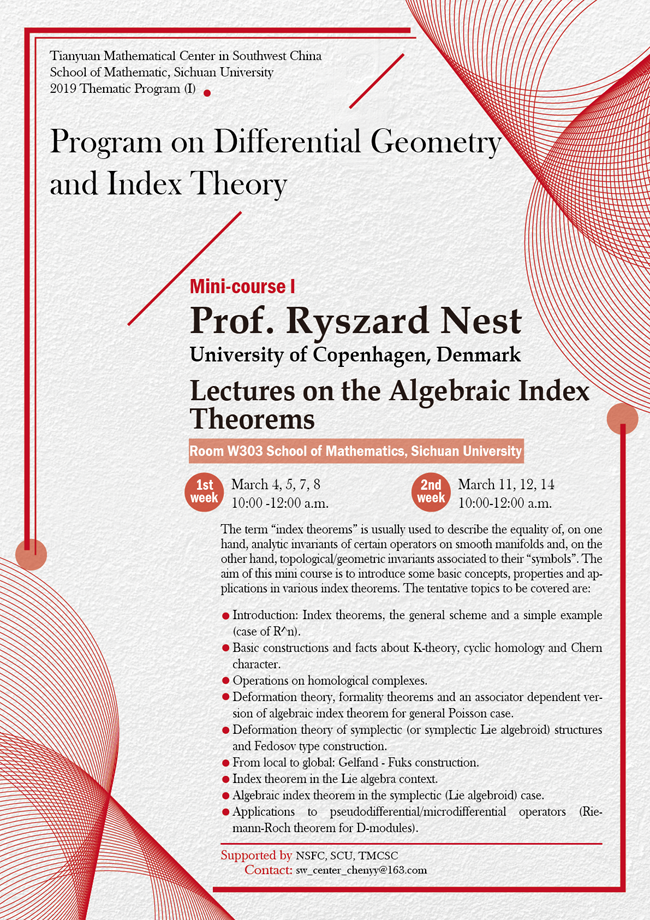2019 Thematic Program (I)

### Lectures on the Algebraic Index Theorems

#### W303  School of Mathematics, Sichuan University#### SPEAKER

Ryszard Nest (University of Copenhagen, Denmark)

#### abstract

The term “index theorems” is usually used to describe the equality of, on one hand, analytic invariants of certain operators on smooth manifolds and, on the other hand, topological/geometric invariants associated to their “symbols”. The aim of this mini course is to introduce some basic concepts, properties and applications in various index theorems. The tentative topics to be covered are:

1. Introduction: Index theorems, the general scheme and a simple example (case of R^n).

2. Basic constructions and facts about K-theory, cyclic homology and Chern character.

3. Operations on homological complexes.

4. Deformation theory, formality theorems and an associator dependent version of algebraic index theorem for general Poisson case.

5. Deformation theory of symplectic (or symplectic Lie algebroid) structures and Fedosov type construction.

6. From local to global: Gelfand - Fuks construction.

7. Index theorem in the Lie algebra context.

8. Algebraic index theorem in the symplectic (Lie algebroid) case.

9. Applications to pseudodifferential/microdifferential operators (Riemann-Roch theorem for D-modules).

#### ORGANIZERD BY

An-Min Li (Chair) (Sichuan University)

Bohui Chen (Sichuan University)

Xiaojun Chen (Sichuan University)

Farkhod Eshmatov (Sichuan University)

Wenchuan Hu (Sichuan University)

Raphael Ponge (Sichuan University)

#### SUPPORTED BY

Natural Science Foundation of China

School of Mathematics, Sichuan University

Tianyuan Mathematical Center in Southwest China

#### VIDEOS

• Lectures on the Algebraic Index Theorems I
• 10:00 - 12:00, 2019-03-04 at W303 School of Mathematics
• Ryszard Nest (University of Copenhagen, Denmark)
• Lectures on the Algebraic Index Theorems II
• 10:00 - 12:00, 2019-03-05 at W303 School of Mathematics
• Ryszard Nest (University of Copenhagen, Denmark)
• Lectures on the Algebraic Index Theorems III
• 10:00 - 12:00, 2019-03-07 at W303 School of Mathematics
• Ryszard Nest (University of Copenhagen, Denmark)
• Lectures on the Algebraic Index Theorems IV
• 10:00 - 12:00, 2019-03-08 at W303 School of Mathematics
• Ryszard Nest (University of Copenhagen, Denmark)
• Lectures on the Algebraic Index Theorems VII
• 10:00 - 12:00, 2019-03-14 at W303 School of Mathematics
• Ryszard Nest (University of Copenhagen, Denmark)